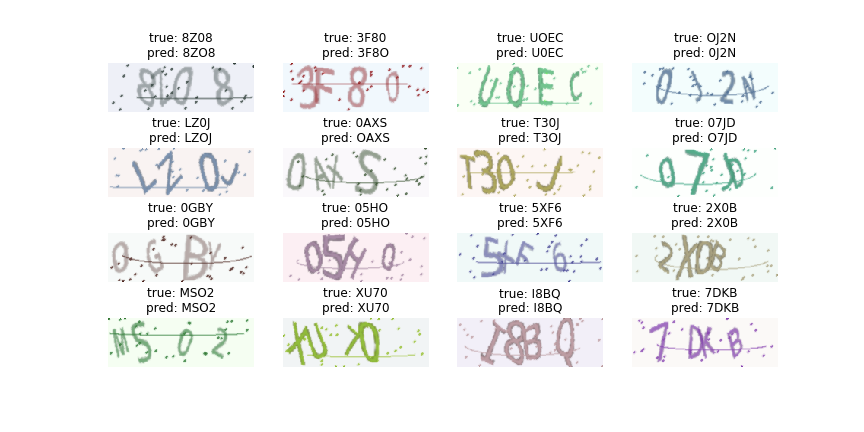# 如何使用 TensorBoard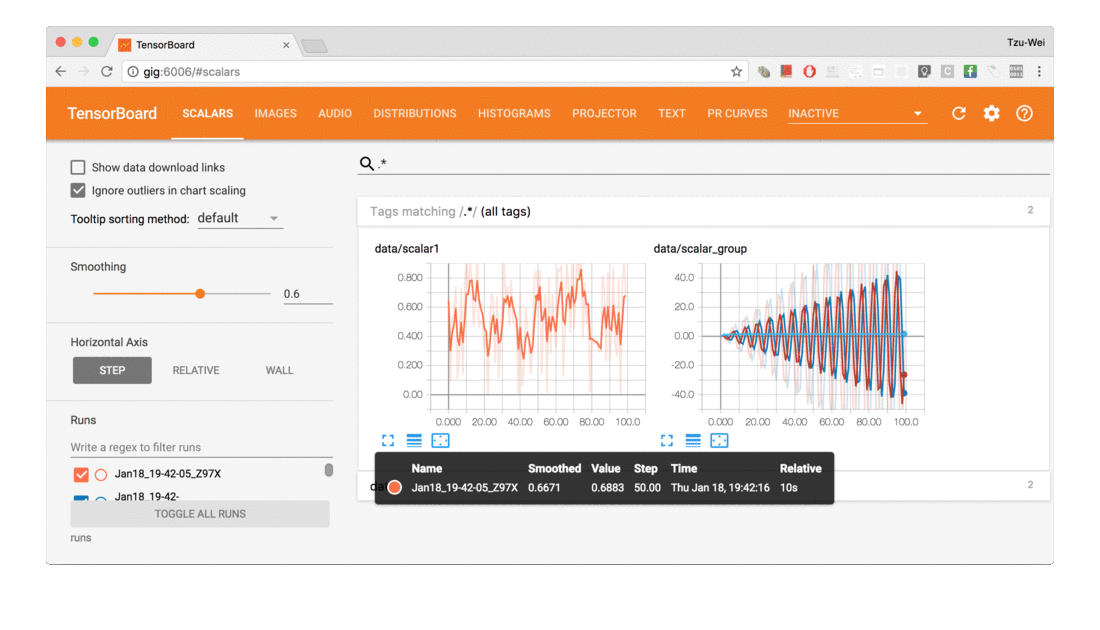## 安装

``````pip install tensorboard tensorboardx
``````

## 使用

• 可视化模型结构
• 按 epoch 可视化 loss、acc
• 按 batch 可视化 loss、acc

• 在代码中对需要可视化的地方添加写出代码
• 打开 TensorBoard 服务

### 创建文件对象（writer）

``````import time
from tensorboardX import SummaryWriter

now = time.strftime("%Y%m%d_%H%M%S", time.localtime())
model_name = f'crnn_{now}'
print(model_name)
writer = SummaryWriter(f'logs/{model_name}')
``````

``````logs
└── crnn_20190630_200056
├── epoch
│   ├── acc
│   │   ├── train
│   │   │   └── events.out.tfevents.1561896176.ypw-black
│   │   └── valid
│   │       └── events.out.tfevents.1561896176.ypw-black
│   ├── error_rate
│   │   ├── train
│   │   │   └── events.out.tfevents.1561896176.ypw-black
│   │   └── valid
│   │       └── events.out.tfevents.1561896176.ypw-black
│   └── loss
│       ├── train
│       │   └── events.out.tfevents.1561896176.ypw-black
│       └── valid
│           └── events.out.tfevents.1561896176.ypw-black
└── events.out.tfevents.1561896056.ypw-black
``````

### 打开 TensorBoard 服务

``````tensorboard --logdir=logs
``````

### 可视化模型结构

``````model = Model(n_classes, input_shape=(3, height, width))
inputs = torch.zeros((1, 3, height, width))
``````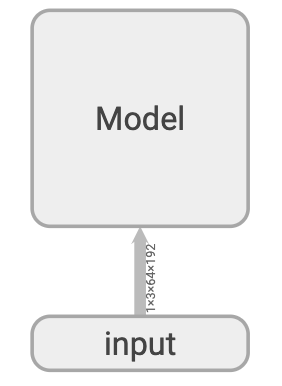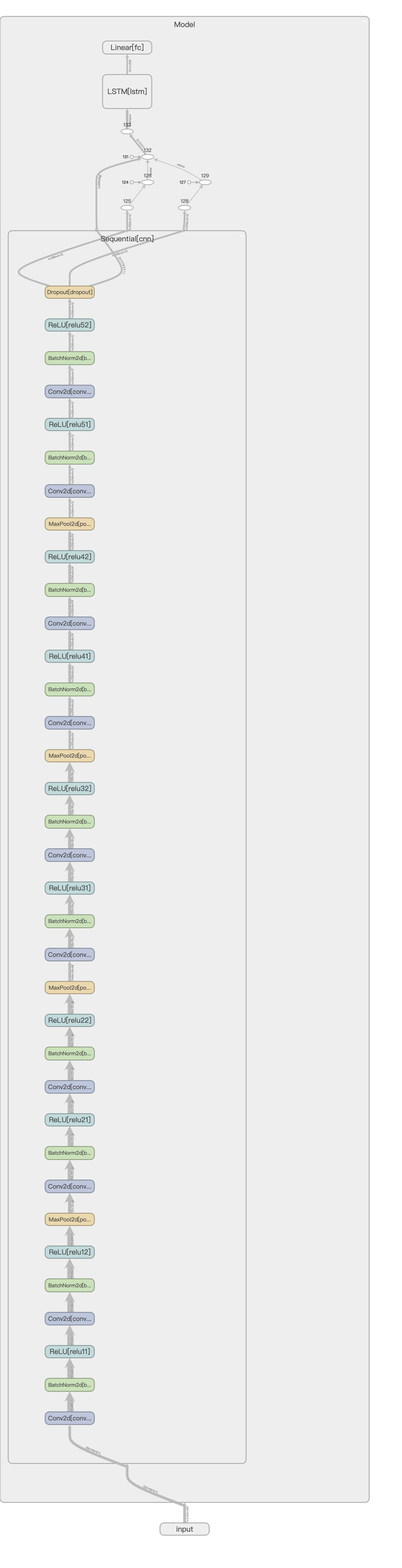### 记录标量（scalar）

``````def train(model, optimizer, epoch, dataloader, writer):
# ...
for batch_index, (data, target) in enumerate(pbar):
# ...
output = model(data)
loss = criterion(output, target)
acc = calc_acc(target, output)
# ...
iteration = (epoch - 1) * len(dataloader) + batch_index
``````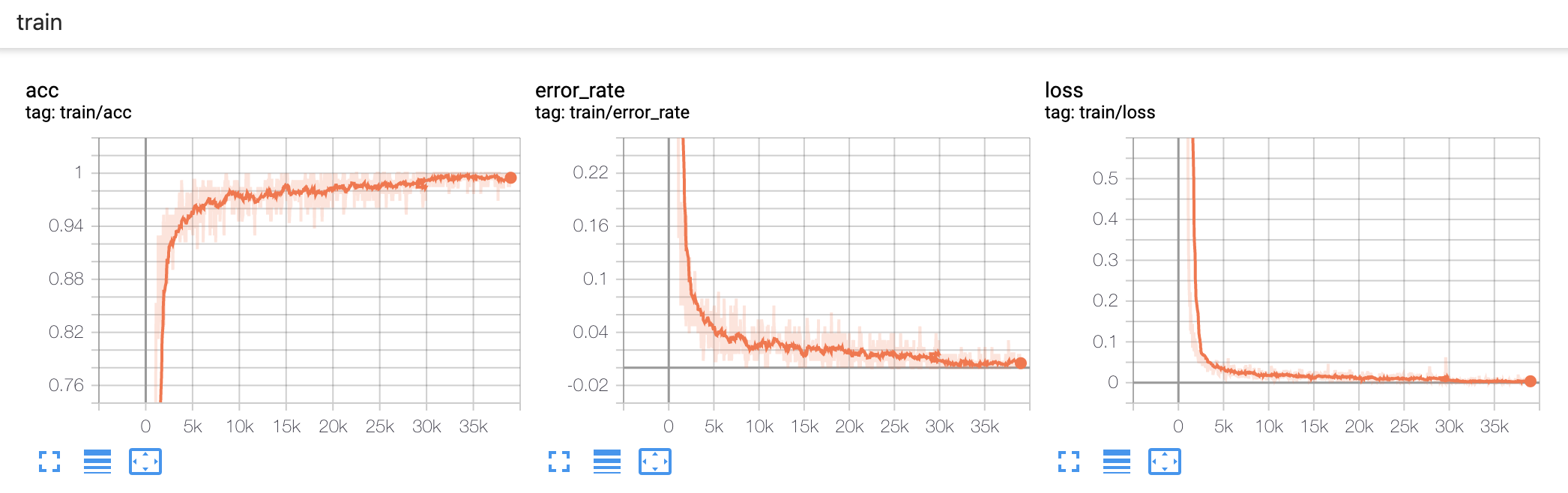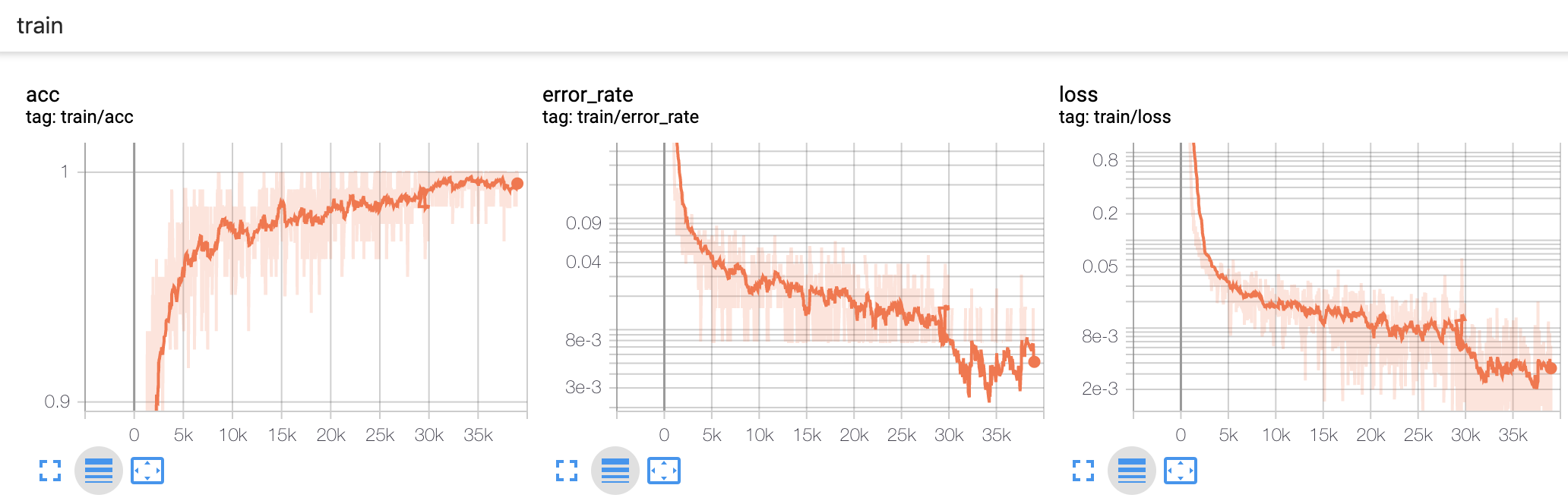### 记录多个标量（scalars）

``````for epoch in range(1, epochs + 1):
train_loss, train_acc = train(model, optimizer, epoch, train_loader, writer)
valid_loss, valid_acc = valid(model, epoch, valid_loader, writer)

writer.add_scalars('epoch/loss', {'train': train_loss, 'valid': valid_loss}, epoch)
writer.add_scalars('epoch/acc', {'train': train_acc, 'valid': valid_acc}, epoch)
writer.add_scalars('epoch/error_rate', {'train': 1 - train_acc, 'valid': 1 - valid_acc}, epoch)
``````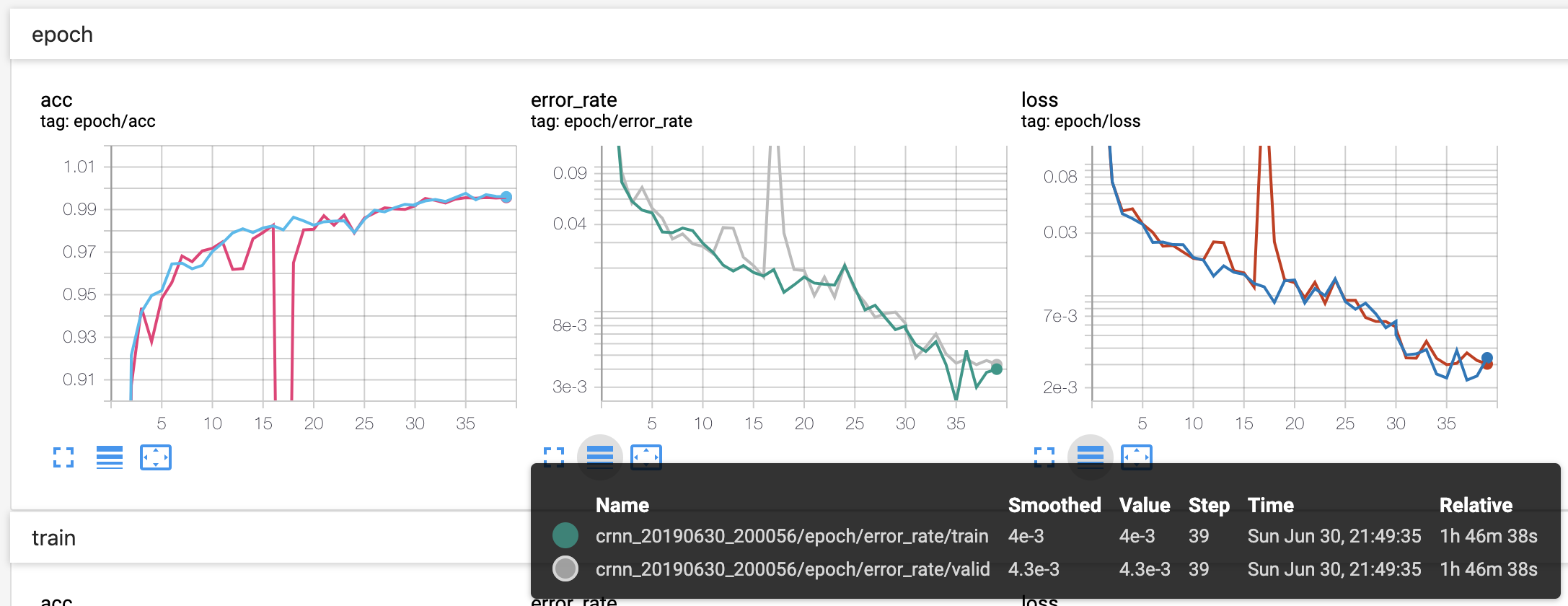### 记录图像（images）

``````# draw badcase
output_argmax = output.detach().permute(1, 0, 2).argmax(dim=-1)
loss = F.ctc_loss(output_log_softmax, target, input_lengths, target_lengths, reduction='none')
hard_sample = np.argsort(loss.detach().cpu().numpy())[::-1]
data = data.cpu()
target = target.cpu()

nrow = 4
ncol = 4
fig = plt.figure(figsize=(12, 6))
for i, index in enumerate(hard_sample[:nrow*ncol]):
plt.subplot(ncol, nrow, i+1)
plt.axis('off')
plt.imshow(data[index].numpy().transpose(1, 2, 0))
s = f'true: {decode_target(target[index])}\npred: {decode(output_argmax[index])}'
plt.title(s)

fig.canvas.draw()
image = np.array(fig.canvas.renderer.buffer_rgba())
plt.close()
``````

OCR 问题既需要图像，又需要文本，所以这里我们使用 matplotlib 绘制图像，然后得到矩阵比较方便。如果你只想拼接多个图像，可以使用 `from torchvision.utils import make_grid` 函数。